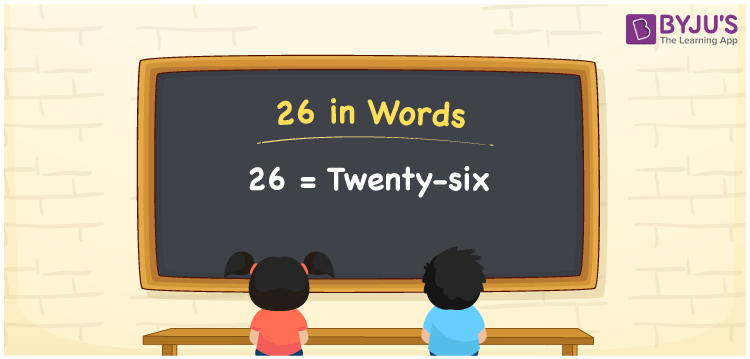# 26 in Words

Twenty-six is the word for the number 26. The number name of 26 is frequently written using place values such as ones, tens, hundreds, and so on. As a result, when writing the number 26 in words, the place value table comes in handy. For instance, you can write “I invested Rs. Twenty-six on a slice of cake” if you invested Rs. 26 on a slice of cake. Here, we will learn how to spell 26 in English words as well as how to express the numerical name 26 in this topic.

 26 in Words: Twenty-six Twenty-six in Numerical Form: 26

## 26 in English Words## How to Write 26 in Words?

To write the number 26 in words, identify the place value of each digit. A place value chart for the number 26 is shown below:

 Tens Ones 2 6

Following is the expanded form for the number 26:

= 2 × Ten + 6 × One

= 2 × 10 + 6 × 1.

= 20 + 6

= 26

= Twenty-six

Hence, 26 in words is twenty-six.

After 25 but before 27, the natural number 26 appears.

26 in words – Twenty-six

Is 26 an odd number? – No

Is 26 an even number? – Yes

Is 26 a perfect square number? – No

Is 26 a perfect cube number? – No

Is 26 a prime number? – No

Is 26 a composite number? – Yes

## Frequently Asked Questions on 26 in Words

Q1

### How to write 26 in words?

26 in words is twenty-six.

Q2

### Compute 30 – 4, and express in words.

Simplifying 30 – 4, we get 26. Hence, 26 in words is twenty-six.

Q3

### Convert twenty-six into numbers.

Twenty-six in numbers is 26.